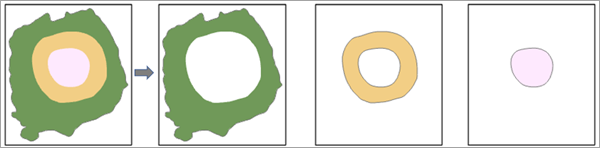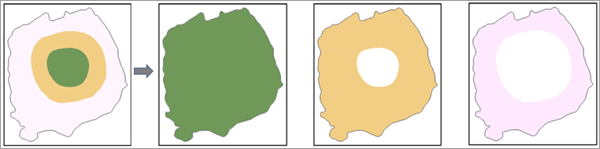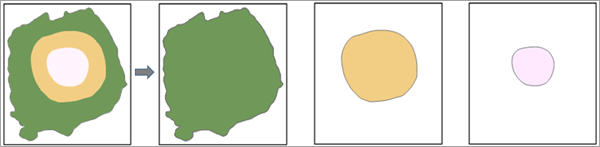# Contour (3D Analyst)

## Summary

Creates a feature class of contours from a raster surface.

## Usage

• Contours do not extend beyond the spatial extent of the raster, and they are not generated in areas of NoData; therefore, adjacent contour inputs should first be edgematched into a continuous feature dataset. As an alternative to edgematching, you can merge the adjacent rasters before computing contours.

However, the contour polygons are extrapolated to the outer edge of the raster when Contour type is not equal to Contour.

• Contours can be generated in areas of negative raster values. The contour values will be negative in such areas. Negative contour intervals are not allowed.

• If you have the ArcGIS Spatial Analyst extension available, smoother but less accurate contours can be obtained by preprocessing the input raster with a Focal Statistics operation with the Mean option or the Filter tool with the Low option.

• A base contour is used, for example, to create contours every 15 meters, starting at 10 meters. In this case, 10 is used for the base contour and 15 is the contour interval. The values to be contoured are 10, 25, 40, 55, and so on.

• Specifying a base contour does not prevent contours from being created above or below that value.

• Contour type is used to produce either contour lines or polygons. For example, if you have a raster with values between 0 and 575 and your contour interval is 250, the following are the various output feature classes that will be created. The actual output is presented, as well as the individual component polygons separately.

• CONTOUR—Lines at 250 and 500
• CONTOUR_POLYGON—Nonoverlapping polygons between 0-250, 250-500, and 500-575 (Fig. 1)• CONTOUR_SHELL—Overlapping polygons between 0-575, 0-500, and 0-250 (Fig. 2)• CONTOUR_SHELL_UP—Overlapping polygons between 0-575, 250-575, and 500-575 (Fig. 3)• The Maximum vertices per feature parameter can be used to subdivide a feature. This should only be used when output features would contain a very large number of vertices (many millions). This parameter produces similar output to that created by the Dice tool.

This parameter is intended as a way to subdivide extremely large features that can cause issues later on, for example, when storing, analyzing, or drawing the features.

Choosing a limit is dependent on the available memory on the machine where the tool is being run and the size of the feature (larger features require more memory). Most modern machines running 64-bit software do not typically have issues with individual features containing hundreds of thousands or millions of vertices. If you do encounter any problems, setting this parameter to a large value, such as 1 million, may alleviate issues due to the size of a feature.

The vertex limit parameter can be used to subdivide a feature. This should only be used when output features would contain a very large number of vertices (many millions).

• By default, this tool will take advantage of multicore processors. The maximum number of cores that can be used is four.

To use fewer cores, use the parallelProcessingFactor environment setting.

• If Extent is specified in the environment setting and the lower-left corner of the output extent does not match any cell corner of the input raster, during processing, a shift of the cell alignment of the input raster will occur to match the specified extent. This shift will trigger a resampling of the input raster using the Bilinear method. Consequently, the output features will shift as well, and the resultant output features may not overlay the original input raster exactly. You can avoid this shift by using the input raster as the Snap Raster in the environment.

## Syntax

`Contour(in_raster, out_polyline_features, contour_interval, {base_contour}, {z_factor}, {contour_type}, {max_vertices_per_feature})`
 Parameter Explanation Data Type in_raster The input surface raster. Raster Layer out_polyline_features The output contour features. Feature Class contour_interval The interval, or distance, between contour lines.This can be any positive number. Double base_contour(Optional) The base contour value.Contours are generated above and below this value as needed to cover the entire value range of the input raster. The default is zero. Double z_factor(Optional) The unit conversion factor used when generating contours. The default value is 1.The contour lines are generated based on the z-values in the input raster, which are often measured in units of meters or feet. With the default value of 1, the contours will be in the same units as the z-values of the input raster. To create contours in a different unit than that of the z-values, set an appropriate value for the z-factor. Note that it is not necessary to have the ground x,y and surface z-units be consistent for this tool.For example, if the elevation values in your input raster are in feet, but you want the contours to be generated based on units of meters, set the z-factor to 0.3048 (since 1 foot = 0.3048 meter). For another example, consider an input raster in WGS_84 geographic coordinates and elevation units of meters for which you want to generate contour lines every 100 feet with a base of 50 feet (so the contours will be 50 ft, 150 ft, 250 ft, and so on). To do this, set the contour_interval to 100, the base_contour to 50, and the z_factor to 3.2808 (since 1 meter = 3.2808 feet). Double contour_type(Optional) Specifies the type of output. The output can represent the contours as either lines or polygons. There are several options for polygons. CONTOUR —A polyline feature class of contours (isolines). This is the default.CONTOUR_POLYGON —A polygon feature class of filled contours.CONTOUR_SHELL —A polygon feature class in which the upper bound of the polygon increases cumulatively by the interval value. The lower bound remains constant at the raster minimum.CONTOUR_SHELL_UP —A polygon feature class in which the lower bound of the polygon increases cumulatively, from the raster minimum, by the interval value. The upper bound remains constant at the raster maximum. String max_vertices_per_feature(Optional) The vertex limit when subdividing a feature. This should only be used when output features contain a very large number of vertices (many millions). This parameter is intended as a way to subdivide extremely large features that can cause issues later on, for example, when storing, analyzing, or drawing the features.If left empty, the output features will not be split. The default is empty. Long

## Code sample

Contour example 1 (Python window)

This example creates contours from an Esri Grid raster and outputs them as a shapefile.

``````import arcpy
from arcpy import env
env.workspace = "C:/data"
arcpy.Contour_3d("elevation", "C:/output/outcontours.shp", 200, 0)``````
Contour example 2 (stand-alone script)

This example creates contours from an Esri Grid raster and outputs them as a shapefile.

``````# Name: Contour_3d_Ex_02.py
# Description: Creates contours or isolines from a raster surface.
# Requirements: 3D Analyst Extension

# Import system modules
import arcpy
from arcpy import env

# Set environment settings
env.workspace = "C:/data"

# Set local variables
inRaster = "elevation"
contourInterval = 200
baseContour = 0
outContours = "C:/sapyexamples/output/outcontours02.shp"

# Execute Contour
arcpy.Contour_3d(inRaster, outContours, contourInterval, baseContour)``````

## Licensing information

• Basic: Requires 3D Analyst or Spatial Analyst
• Standard: Requires 3D Analyst or Spatial Analyst
• Advanced: Requires 3D Analyst or Spatial Analyst